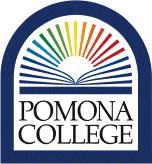# CSC 081 Computability and Logic

Computability refers to the study of the mathematical foundations of computation: what is an appropriate mathematical model of a computer, what types of computations are possible in the model, what types are not, the inherent complexity of certain computations and so forth. Perhaps surprisingly, many concepts from the theory of computation have become of fundamental importance in other areas of computer science, such as computational linguistics, compiler design, hardware design, object-oriented design, artificial intelligence, and even the syntax of the UNIX grep and awk commands.

In this course we will investigate the interaction between various models of computation. Along the way the intimate connection between computation and language recognition will be developed. We will study several classes of abstract machine including finite automata, push-down automata and Turing machines along with several classes of languages such as regular and context-free languages. In addition we will examine some of those problems, such as the Halting Problem, which are not amenable to computer solution.

We will also investigate the use of formal logics in computer science, defining a variety of logics and examining their strengths and weaknesses in expressiveness and the difficulty of creating proofs. We will examir logics that are particularly useful in proving programs (in both software and hardware) correct.

By the end of this course, you should be able to:

1. Describe and use formal systems to model real phenomena
2. Understand the differences between and use of formal syntax and semantics.
3. Understand and be able to write proofs in several formal logic systems.
4. Understand the importance of the notions of soundness and completeness for formal logics.
5. Be able to use formal logics to prove the correctness of computer programs.
6. Understand the uses of and differences between finite automata, pushdown automata, and Turing machine models of computation.
7. Understand and use the correspondence between formal grammars and the machines that can recognize them.
8. Understand the Church-Turing thesis and several models of universal computation.
9. Understand and be able to show that a variety of interesting problems involving computation are undecidable.

To simplify printing of the information about CSC 081 found on these pages, a single page on which all the information about CSC 081 is grouped together is also available.